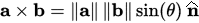# RD Sharma Solutions Class 12 Chapter 25 Vector or Cross Product

## RD Sharma Solutions for Class 12 Maths Chapter 25 – Free PDF Download

The RD Sharma Class 12 Solutions Chapter 25 Vector or Cross Product is given here. The complete RD Sharma Solutions for Class 12 for this chapter are given chapter-wise in a detailed manner so that students can access all the solutions in the same pattern and sequence as in the RD Sharma textbooks. These solutions are curated by subject experts at BYJU’S.

RD Sharma Solutions is a must-have resource for students while solving the exercise problems of the Class 12 RD Sharma Textbook. Students can access the RD Sharma Solutions both online and as downloadable PDF versions according to the needs of the students. Further, the solutions are in accordance with the latest CBSE guidelines and marking schemes to help students fetch high marks.

### Key Insights in RD Sharma Class 12 Solutions Chapter 25 Vector or Cross Product

Let ‘a’ and ‘b’ be two non-zero parallel vectors. Then the vector product a * b, in that order, is defined as a vector whose magnitude is |a| |b| sin θ, where θ is the angle between a & b and whose direction is perpendicular to the plane of a & b in such a way that a, b and this direction constitute a right-handed system.

The cross product of two vectors a and b is defined only in three-dimensional space and is denoted by a × b. The Vector or Cross Product is defined by the formula:Check the RD Sharma Solution for Class 12 Chapter 25: Vector or Cross Product given in a detailed way in the below-mentioned table.

 Vector or Cross Product Class 12 RD Sharma Exercises Vector or Cross Product Exercise 25.1